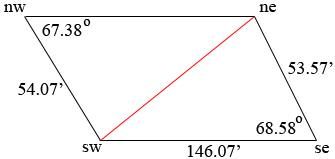SEARCH HOMEMath Central Quandaries & QueriesQuestion from Penny: Property has 3 measurements and 2 angles and I want the altitude at a particular point. Corners are sw, nw, ne and se. 1 want the 4th side measurement confirmed (we think 146.07') and the altitude measurement at 25' from sw (on the sw-se side) The angles provided are interior at nw 67.38 degrees and the opposing corner se interior appears to be 68.58 degrees. the 3 sides with measurements are: sw to nw=54.07'. sw to se 146.07' and se to ne 53.57'. If you would help, we would really appreciate it. Thanks! PennyHi Penny,

I got for the length of the third side to be slightly different than what you got. I got 147.29 feet. Here is what I did.First I let the length of the diagonal from sw to ne be c feet. I then used the Law of Cosines on the triangle with vertices ne, sw and se to get

c2 = 146.072 + 53.572 - 2 × 146.07 × 53.57 ×cos(68.58)

This gave me c = 135.98 feet.

Next I used the Law of Sines on the triangle with vertices ne, nw and sw. If the measure of the angle from sw to ne to nw is θ degrees then

sin(θ)/54.07 = sin(67.38)/135.98

Solving for sin(θ) I got sin(θ) = 0.3670 and then the inverse sine function on my calculator gave θ = 21.53 degrees. Thus the measure of the angle from nw to sw to ne is 180 - 67.38 - 21.53 = 91.09 degrees. Using the Law of Sines again with d the length of the side from ne to nw

sin(67.38)/135.98 = sin(91.09)/d

and solving for d gave 147.29 feet.

HarleyMath Central is supported by the University of Regina and The Pacific Institute for the Mathematical Sciences.